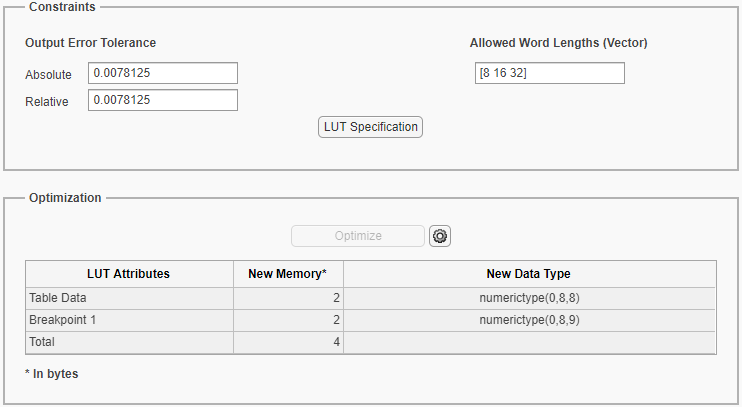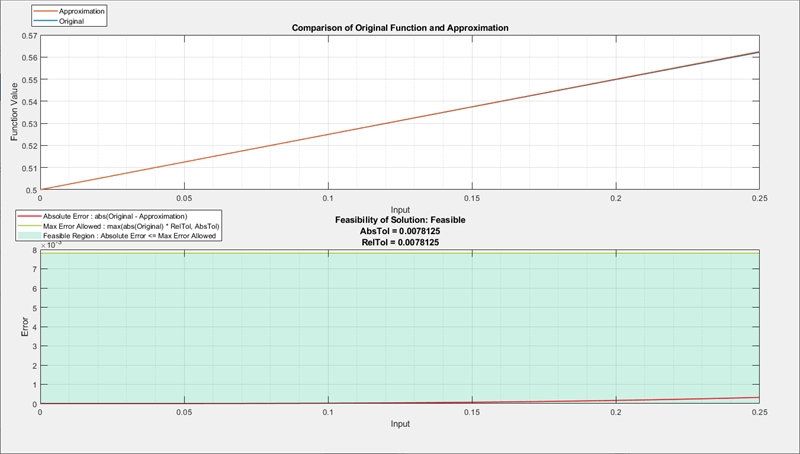Generate an Optimized Lookup Table as a MATLAB Function

This example shows how to approximate y = 1/(1+exp^(-x)) as a MATLAB® function lookup table using the Lookup Table Optimizer.

1. To open the Lookup Table Optimizer, on the Simulink® Apps tab, in the Code Generation gallery, click Lookup Table Optimizer.

2. In the Objective pane of the app, select the source as MATLAB Function Handle. Click Next.

3. In the Setup pane, provide the function handle @(x)(1/(1+exp(-x))).

The attributes populate in the table below. You can manually edit the fields to specify ranges and data types other than those populated. For this example, set Minimum to 0 and Maximum to 0.25.

Click Next.

4. In the Create pane, specify the Output Error Tolerance that is acceptable for your design.

To specify additional properties for the optimized lookup table, click . Change the Solution Type to MATLAB.

After you are satisfied with the constraints and additional options, click . When the optimization is complete, the Lookup Table Optimizer reports the memory of the optimized lookup table. You can edit the constraints and run the optimization again to achieve further memory reduction.Click to view the Results pane.

5. Click to view a plot of the original function output compared to the output of the new optimized lookup table.6. Click to view the lookup table function launched in the MATLAB Command Window.